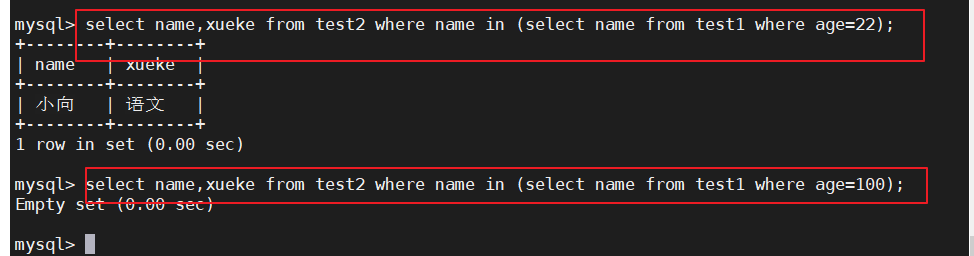# 一、SQL高级语句

## 1、 SELECT

``````select * from test1;
select name from test1;
select name,sex from test1;
``````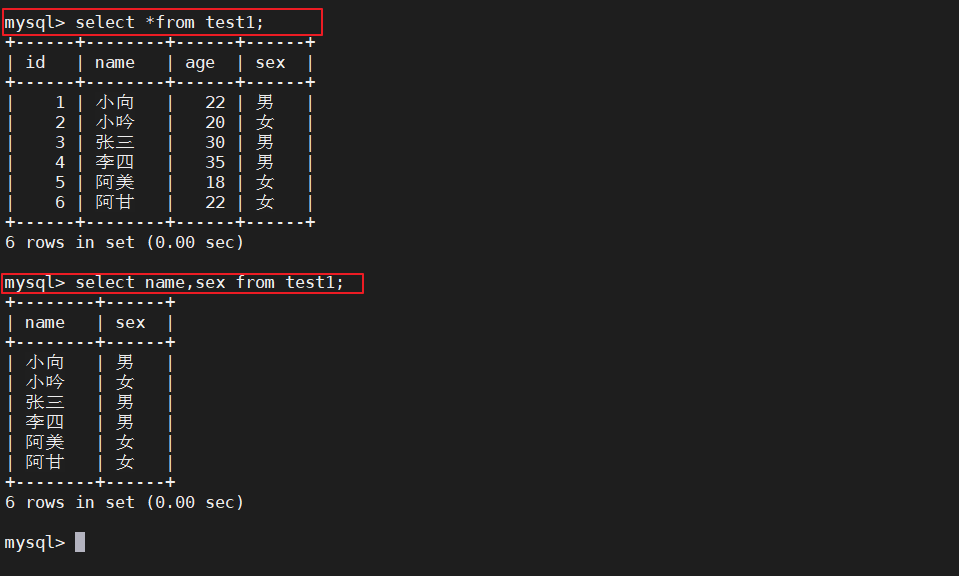## 2、DISTINCT

``````select distinct age from test1;
``````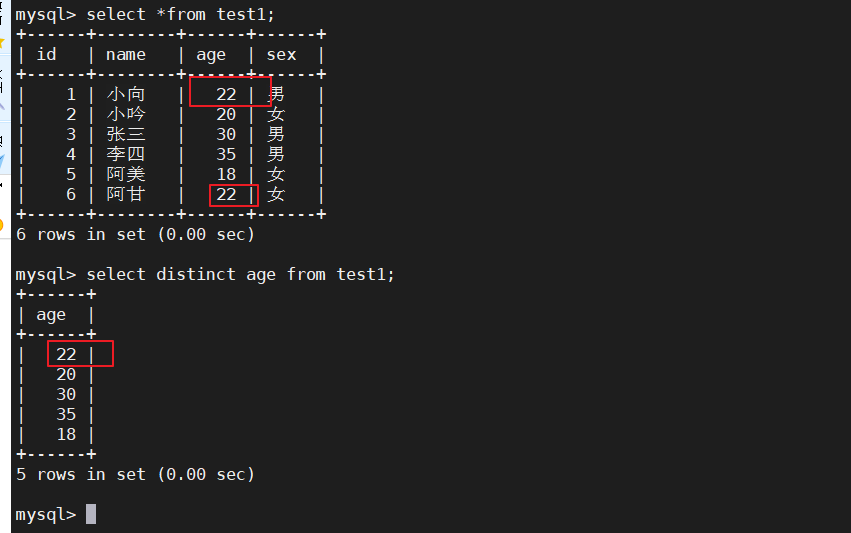## 3、WHERE

``````select name,age from test1 where age < 21;
select name,age from test1 where name='nancy';
``````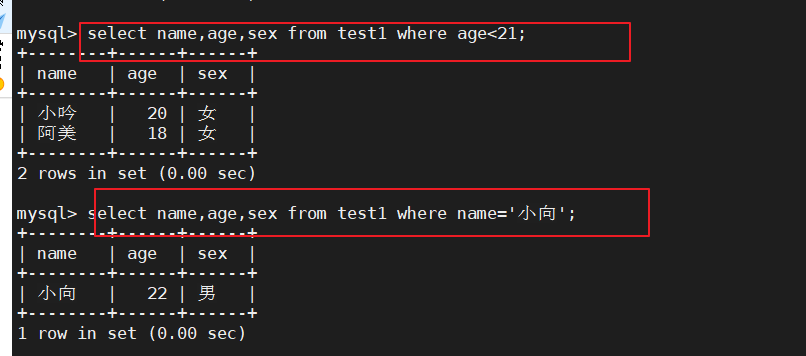## 4 、AND OR

``````select * from test1 where sex='女' and age > 21;
select * from test1 where age > 21 or age < 20;
select * from test1 where (age > 21 and sex='男') or age < 20;
``````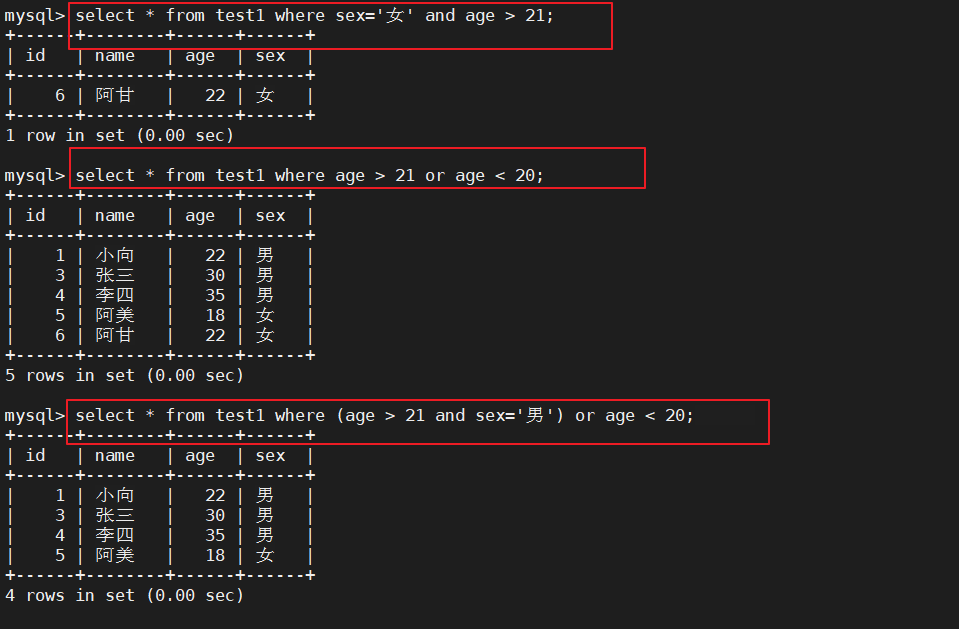## 5、IN

``````select * from test1 where age in (18,20,35);
``````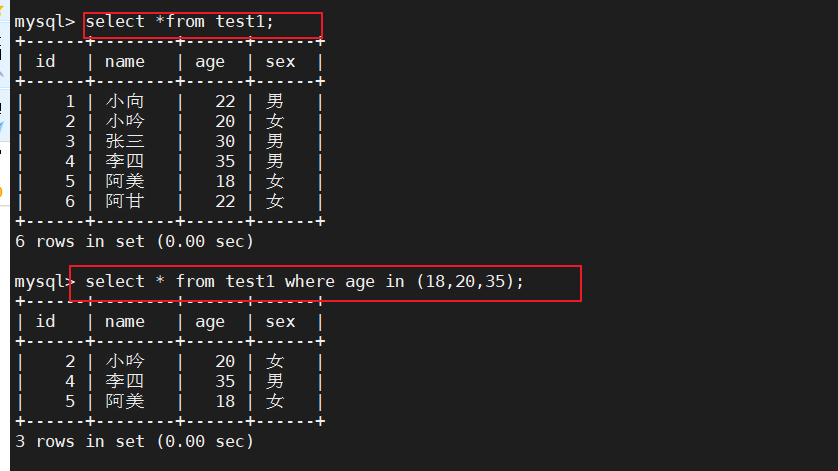## 6、BETWEEN

``````select * from test1 where age between 19 and 30;
``````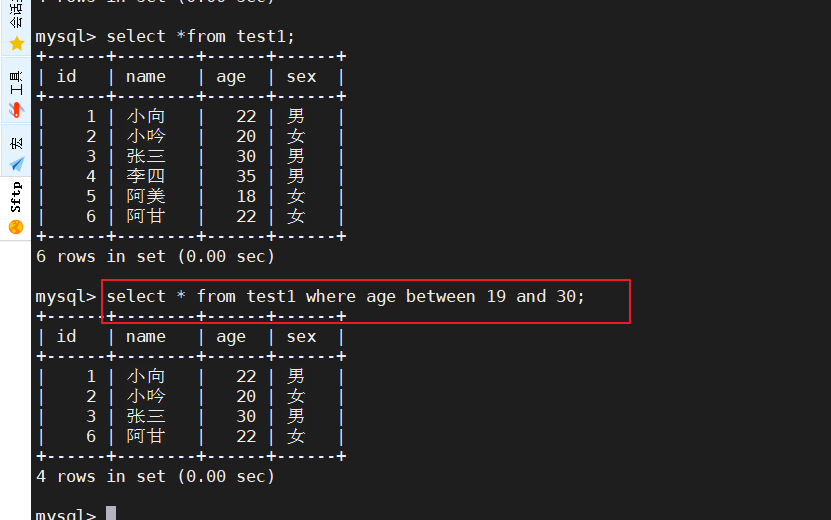## 7 通配符

%百分号表示零个、一个或多个字符（相当于linux中的“*”号）
_下划线表示单个字符（相当于linux中的“?”号）
‘A_Z’所有以 ‘A’ 起头，另一个任何值的字符，且以 ‘Z’ 为结尾的字符串。例如，‘ABZ’ 和 ‘A2Z’ 都符合这一个模式，而 ‘AKKZ’ 并不符合 (因为在 A 和 Z 之间有两个字符，而不是一个字符)。
‘ABC%’所有以 ‘ABC’ 起头的字符串。例如，‘ABCD’ 和 ‘ABCABC’ 都符合这个模式
‘%XYZ’所有以 ‘XYZ’ 结尾的字符串。例如，‘WXYZ’ 和 ‘ZZXYZ’ 都符合这个模式
‘%AN%’所有含有 'AN’这个模式的字符串。例如，‘LOS ANGELES’ 和 ‘SAN FRANCISCO’ 都符合这个模式
‘_AN%’所有第二个字母为 ‘A’ 和第三个字母为 ‘N’ 的字符串。例如，‘SAN FRANCISCO’ 符合这个模式，而 ‘LOS ANGELES’ 则不符合这个模式

## 8 LIKE

``````select * from test1 where name like '小%';
select *from test1 where name like '%小%';
select *from test1 where name like '李_';
``````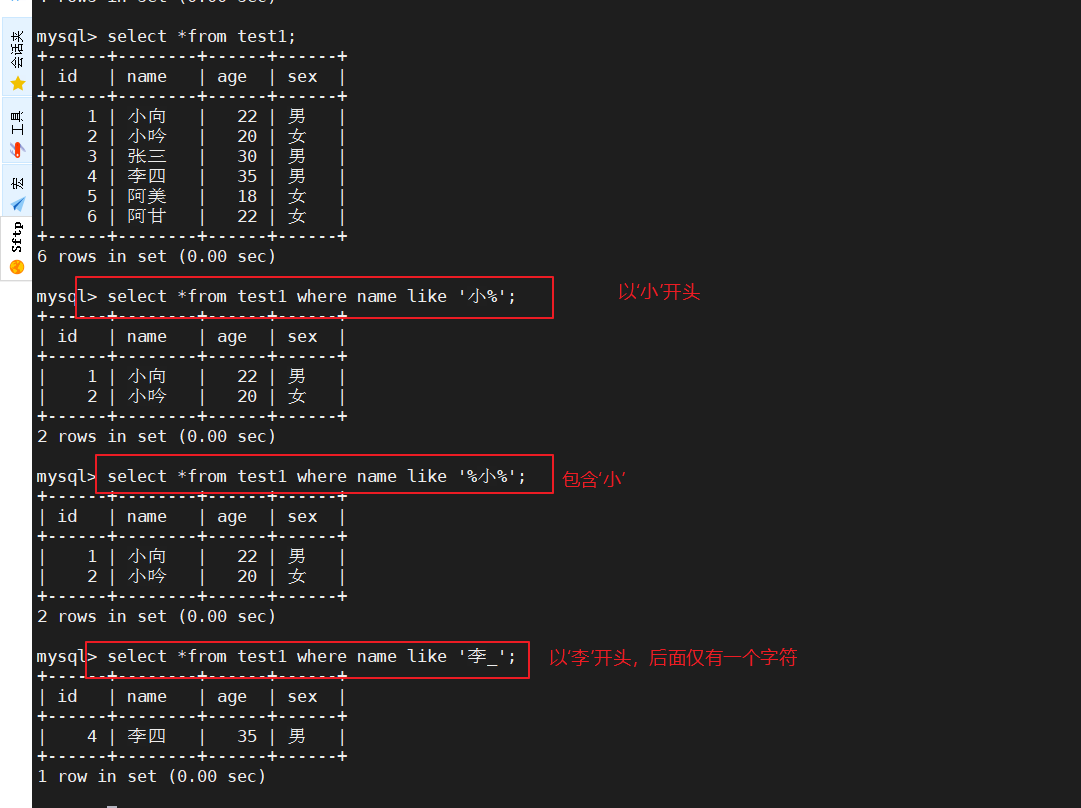## 9 ORDER BY

#ASC 是按照升序进行排序的，是默认的排序方式。

#DESC 是按降序方式进行排序。

``````select *from test1 where sex='男' order by age;
select *from test1 where sex='男' order by age desc;
``````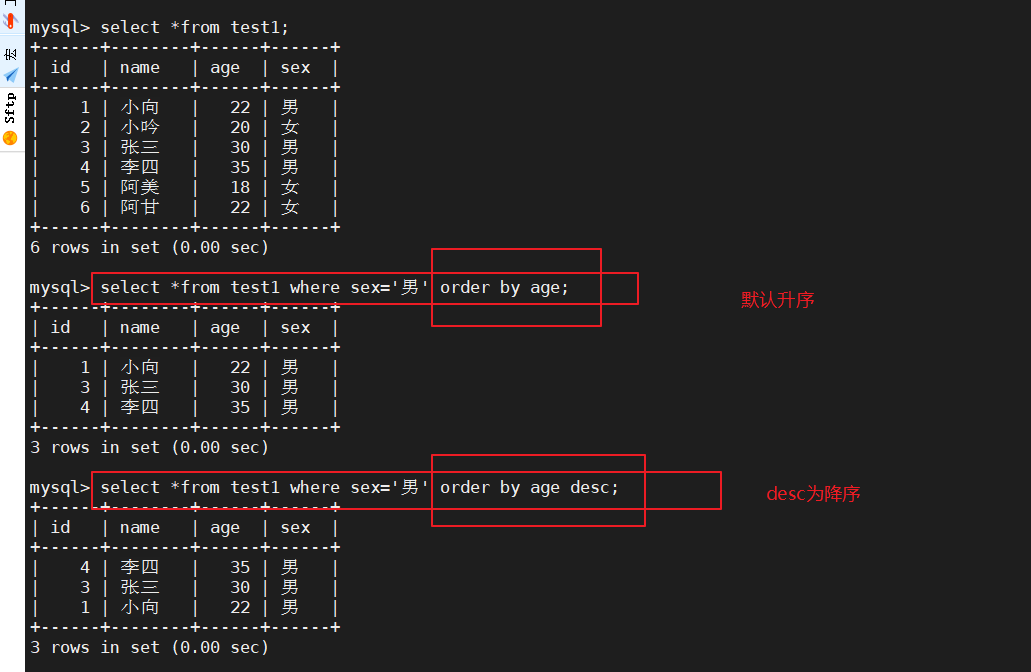# 二、SQL函数

## 1、数学函数：

abs(x)返回 x 的绝对值
rand()返回 0 到 1 的随机数
mod(x,y)返回 x 除以 y 以后的余数
power(x,y)返回 x 的 y 次方
round(x)返回离 x 最近的整数
round(x,y)保留 x 的 y 位小数四舍五入后的值
sqrt(x)返回 x 的平方根
truncate(x,y)返回数字 x 截断为 y 位小数的值
ceil(x)返回大于或等于 x 的最小整数
floor(x)返回小于或等于 x 的最大整数
greatest(x1,x2…)返回集合中最大的值
least(x1,x2…)返回集合中最小的值
``````select abs(-2),rand(),mod(10,3),power(2,3),round(5.66);
``````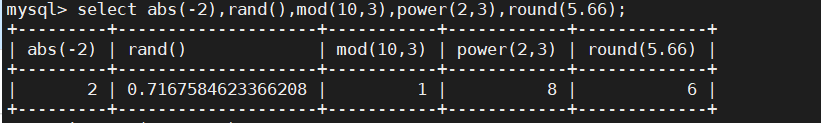``````select round(5.445636,4),sqrt(3),truncate(8.456547,4);
``````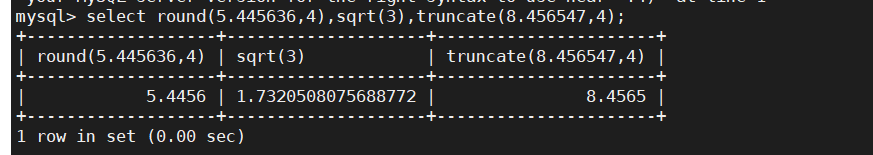## 2 聚合函数：

avg()返回指定列的平均值
count()返回指定列中非 NULL 值的个数
min()返回指定列的最小值
max()返回指定列的最大值
sum(x)返回指定列的所有值之和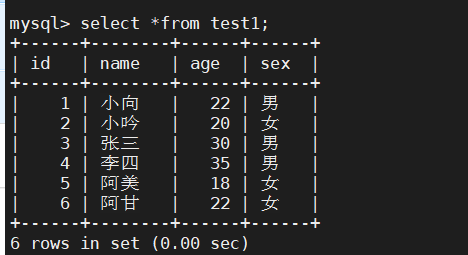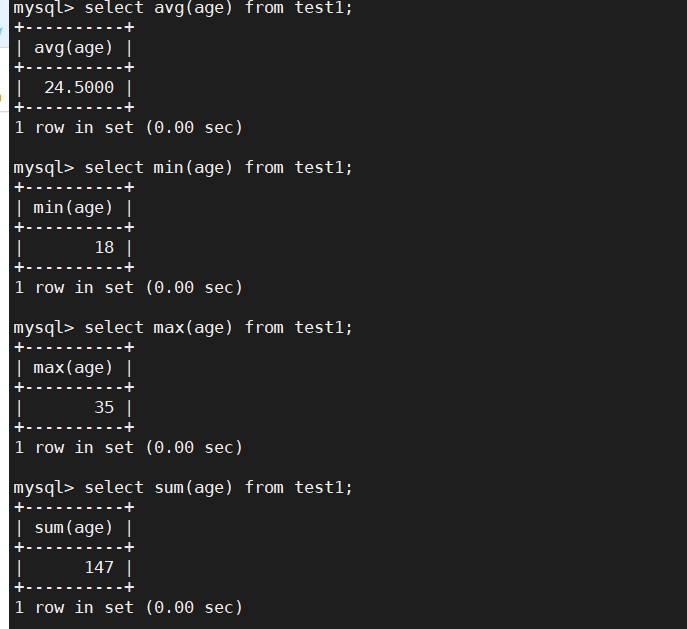count

count(*) 表示包括所有列的行数，不会忽略null值

count(列名) 表示只包括这一列，统计时会忽略null值的行

## 3 字符串函数：

trim()返回去除指定格式的值
concat(x,y)将提供的参数 x 和 y 拼接成一个字符串
substr(x,y)获取从字符串 x 中的第 y 个位置开始的字符串，跟substring()函数作用相同
substr(x,y,z)获取从字符串 x 中的第 y 个位置开始长度为 z 的字符串
length(x)返回字符串 x 的长度
replace(x,y,z)将字符串 z 替代字符串 x 中的字符串 y
upper(x)将字符串 x 的所有字母变成大写字母
lower(x)将字符串 x 的所有字母变成小写字母
left(x,y)返回字符串 x 的前 y 个字符
right(x,y)返回字符串 x 的后 y 个字符
repeat(x,y)将字符串 x 重复 y 次
space(x)返回 x 个空格
strcmp(x,y)比较 x 和 y，返回的值可以为-1,0,1
reverse(x)将字符串 x 反转

## 4 GROUP BY

GROUP BY有个原则，select 后面的所有列中，没有用聚合函数的列，必须出现在GROUP BY后面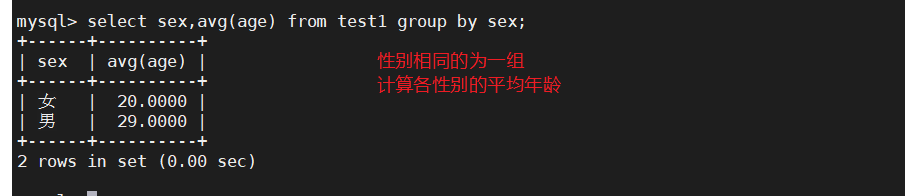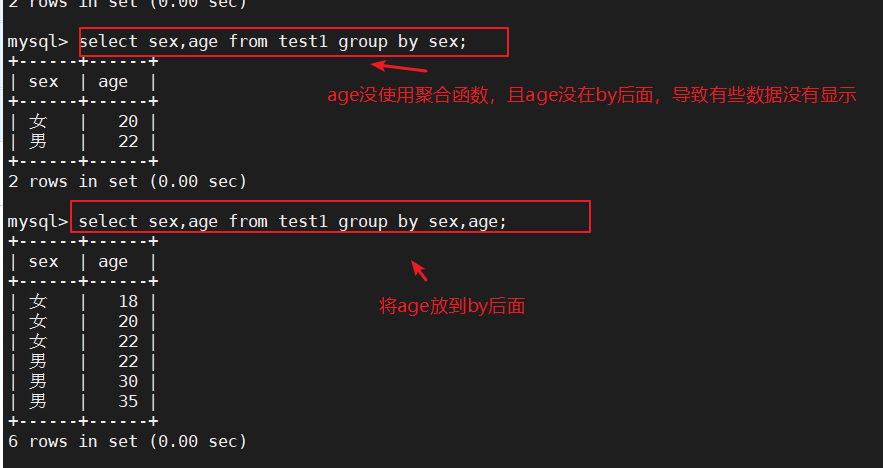## 5 HAVING

HAVING的存在弥补了WHERE关键字不能和聚合函数联合使用的不足，如果被select的只有函数，那就不需要GROUP BY语句

HAVING只是条件过滤，用来筛选前面GROUP BY的结果

``````select sex,avg(age) from test1 group by sex;
select sex,avg(age) from test1 group by sex having avg(age)>25;
``````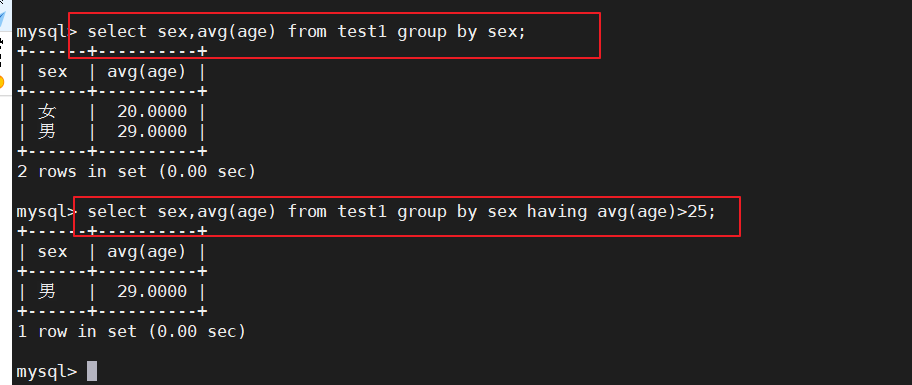## 6 别名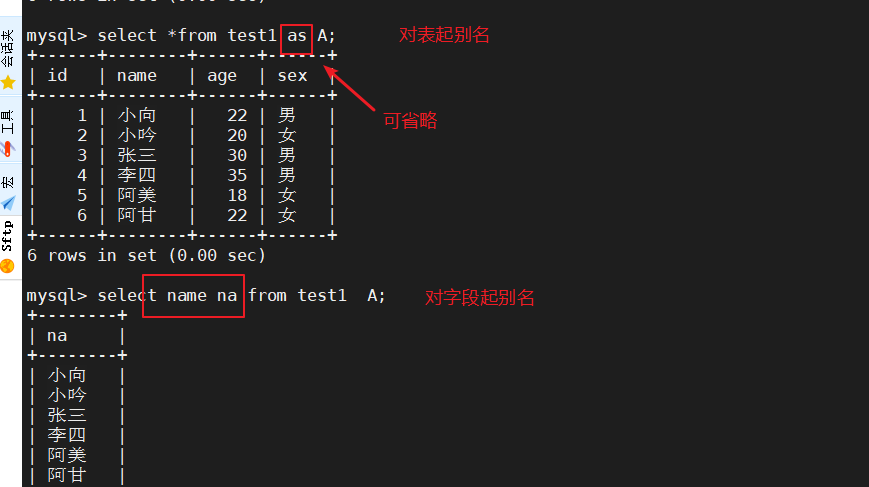## 7 连接查询

### 7.1 inner join(等值相连)

``````select * from test2 A inner join test1 B on A.name = B.name;
``````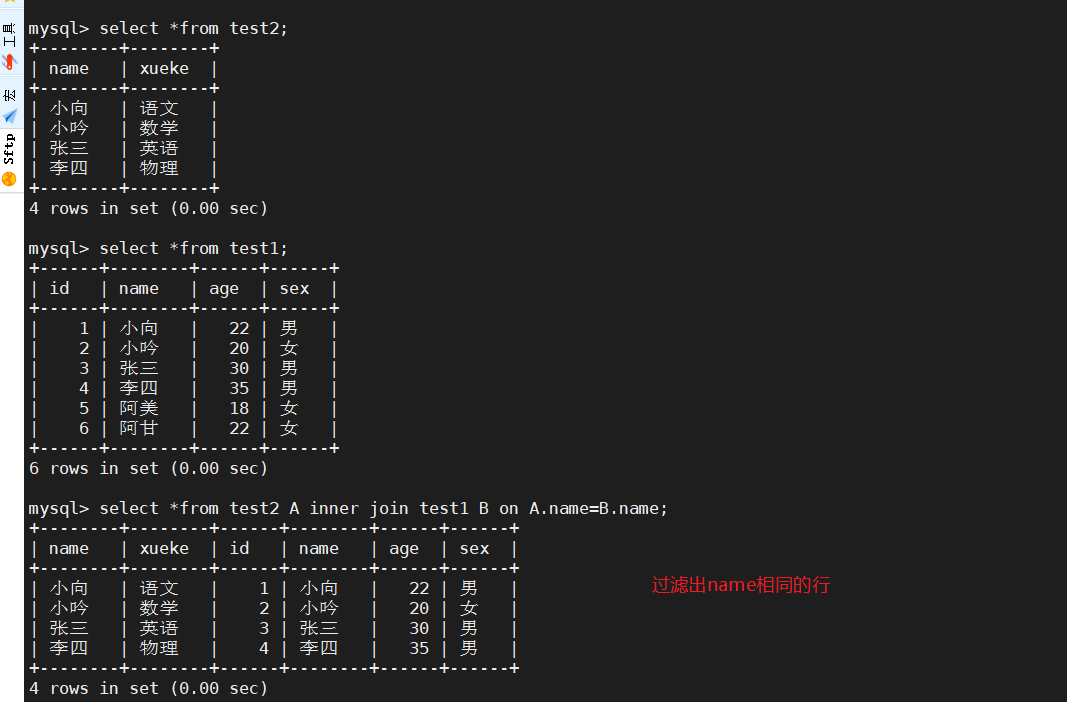### 7.2 left join(左联接)

``````select * from test2 A left join test1 B on A.name = B.name;
``````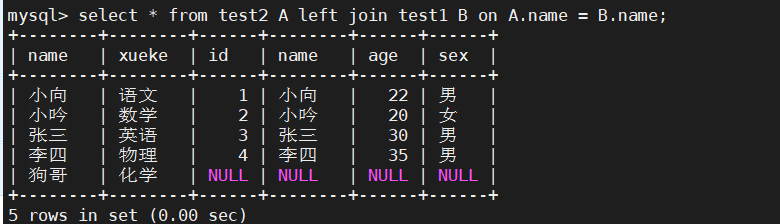### 7.3 right join(右联接)

``````select * from test2 A right join test1 B on A.name = B.name;
``````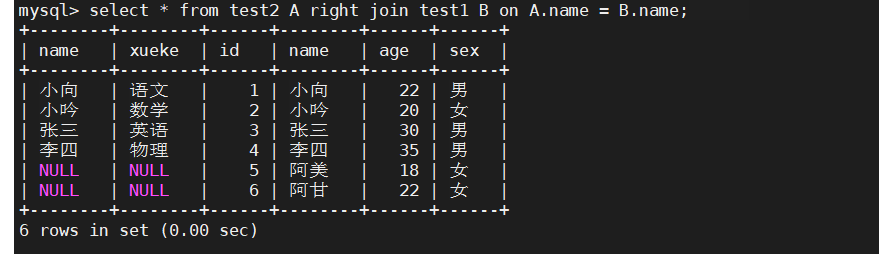## 8 子查询

``````语法：SELECT "字段1" FROM "表格1" WHERE "字段2"  [比较运算符]          #外查询
(SELECT "字段2" FROM "表格2" WHERE "条件");                           #内查询
``````

``````select xueke from test2 where name in (select name from test1 where age=22);
``````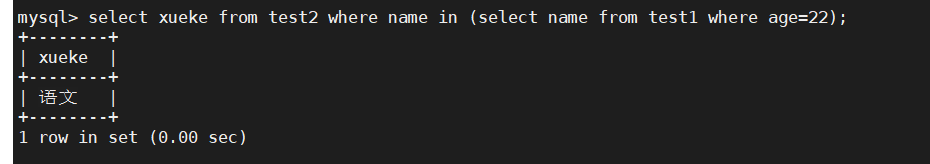## 9 EXISTS

``````select name,xueke from test2 where name in (select name from test1 where age=22);
select name,xueke from test2 where name in (select name from test1 where age=100);
``````